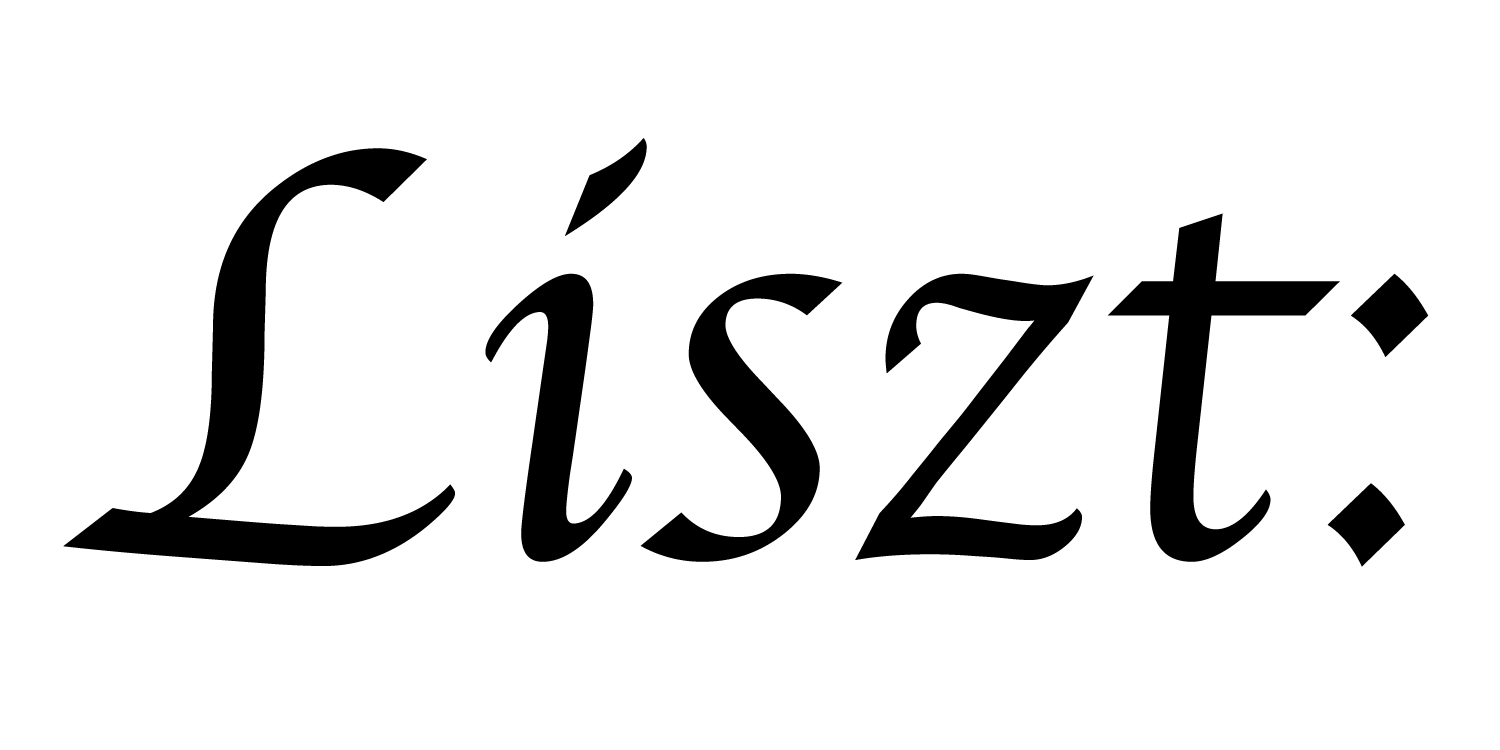# Tutorials

## Introduction

These tutorials provide a tour through all of the basic features of Ebb, sufficient to get started writing your own simulations using the standard domains.

## 01: Hello, 42!

The basics of an Ebb program; print out 42 for each element of a grid.

How to use a domain library to load in a mesh from a file and some very basic statistics and computations on that mesh; We use an octahedron to demonstrate.

## 03: Visualizing Simulations

Basic usage of VDB to generate visual output from Ebb programs; We plot the 6 vertices of the octahedron.

## 04: User-defined Fields and Globals

Defining simulation specific data per-mesh-element (fields) and globally; Using these features we make our octahedron oscillate.

## 05: Accessing Neighbors

How to access data at neighboring elements of the mesh/domain; Now we can write a heat-diffusion on the surface of the Stanford bunny triangle mesh.

## 06: Phases, Reads, Writes, Reductions

A key feature of Ebb is that all functions are safe to parallelize; We explain the rules and show alternative ways of writing the heat diffusion.

## 07: Using Standard Grids

Some features of the standard grid domain; we show how to write heat diffusion on a grid, handling both periodic and normal boundary conditions.

## 08: Relations

Relations are the basic data structure in Ebb that everything else is built out of; we build a torus from scratch and simulate heat diffusion on it.

## 09: Particle-Grid Coupling

How to connect and update the relationship between particles and a grid; we advect tracer particles in an evolving heat gradient.

## Interoperability

These tutorials introduce the features that let Ebb interoperate with C-code, including how to write custom high-performance File I/O libraries. (familiarity with the introduction tutorials is assumed)

## 10: Data Layout Descriptors (DLDs)

DLDs give us raw access to the simulation memory used by Ebb; we use this access to integrate a piece of unsafe code into an Ebb program.

## 11: Calling C-code

DLDs can also be used from C code written entirely outside of Ebb and Lua; we link an unsafe C function into an Ebb simulation.

## 12: File I/O

Using DLDs, we can efficiently load data into and dump data out of an Ebb simulation; we write code to load and write OFF triangle mesh files.

## 18: C Embedding

Ebb is built on Lua and Terra, which makes using the Ebb runtime from a controlling C program as straightforward as library linking.

## Domain Modeling

These tutorials explain the features that are used to write the standard geometric domain libraries; after reading these tutorials, a programmer should be prepared to start developing their own custom geometric domains. (familiarity with the introduction tutorials is assumed)

## 13: Group-By and Query-Loops

Grouping and Querying lets us invert simple relationship between elements; we simulate heat diffusion on a graph encoded with grouping.

## 14: Join Tables

A common pattern that enables us to represent arbitrary graph connectivities; We use it to enable access to the triangles around a vertex.

## 15: Macros

Macros let us hide unintuitive encodings behind more familiar syntax; we clean up the join-table example using macros.

## 16: Grid Relations

How to use relations to represent data from a grid domain; we show how to build a two-scale coupled grid-to-grid domain for simulation.

## 17: Subsets

Subsets let us execute computations for only parts of a domain; we build subsets to let us express boundary conditions in a grid heat diffusion.

a part of the Liszt project and PSAAP II center at Stanford University